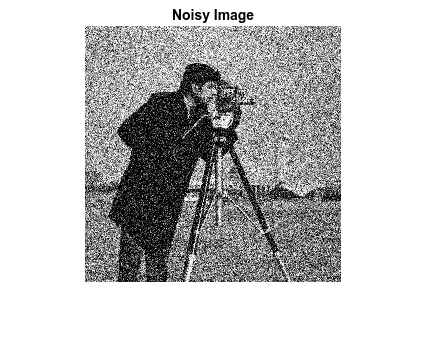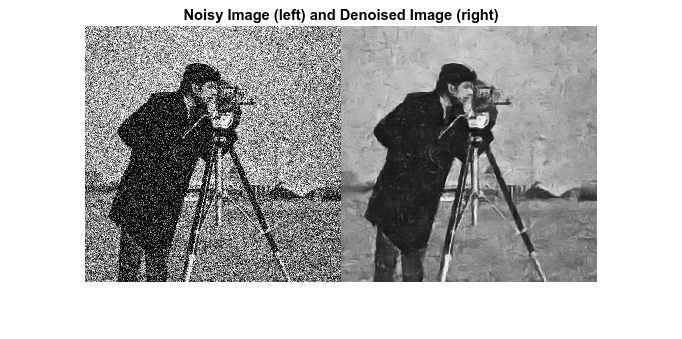Main Content

# Code Generation for Denoising Deep Neural Network

This example shows how to generate CUDA® MEX from MATLAB® code and denoise grayscale images by using the denoising convolutional neural network (DnCNN ). You can use the denoising network to estimate noise in a noisy image, and then remove it to obtain a denoised image.

### Third-Party Prerequisites

Required

This example generates CUDA MEX and has the following third-party requirements.

• CUDA® enabled NVIDIA® GPU and compatible driver.

Optional

For non-MEX builds such as static, dynamic libraries or executables, this example has the following additional requirements.

### Verify GPU Environment

Use the `coder.checkGpuInstall` (GPU Coder) function to verify that the compilers and libraries necessary for running this example are set up correctly.

```envCfg = coder.gpuEnvConfig('host'); envCfg.DeepLibTarget = 'cudnn'; envCfg.DeepCodegen = 1; envCfg.Quiet = 1; coder.checkGpuInstall(envCfg); ```

### Load Noisy Image

Load a noisy grayscale image into the workspace and display the image.

```noisyI = imread('noisy_cameraman.png'); figure imshow(noisyI); title('Noisy Image'); ```### Get Pretrained Denoising Network

Call the `getDenoisingNetwork` helper function to get a pretrained image denoising deep neural network.

```net = getDenoisingNetwork; ```

The `getDenoisingNetwork` function returns a pretrained DnCNN  that you can use to detect additive white Gaussian noise (AWGN) that has unknown levels. The network is a feed-forward denoising convolutional network that implements a residual learning technique to predict a residual image. In other words, DnCNN  computes the difference between a noisy image and the latent clean image.

The network contains 59 layers including convolution, batch normalization, and regression output layers. To display an interactive visualization of the deep learning network architecture, use the `analyzeNetwork` function.

```analyzeNetwork(net); ```

### The `denoisenet_predict` Function

The `denoisenet_predict` entry-point function takes a noisy image input and returns a denoised image by using a pretrained denoising network.

The function loads the network object returned by `getDenoisingNetwork` into a persistent variable mynet and reuses the persistent object on subsequent prediction calls.

```type denoisenet_predict ```
```function I = denoisenet_predict(in) %#codegen % Copyright 2018-2019 The MathWorks, Inc. persistent mynet; if isempty(mynet) mynet = coder.loadDeepLearningNetwork('getDenoisingNetwork', 'DnCNN'); end % The activations methods extracts the output from the last layer. The % 'OutputAs' 'channels' name-value pair argument is used inorder to call % activations on an image whose input dimensions are greater than or equal % to the network's imageInputLayer.InputSize. res = mynet.activations(in, 59,'OutputAs','channels'); % Once the noise is estimated, we subtract the noise from the original % image to obtain a denoised image. I = in - res; ```

Here, the `activations` method is called with the layer numeric index as 59 to extract the activations from the final layer of the network. The `'OutputAs'` `'channels'` name-value pair argument computes activations on images larger than the `imageInputLayer.InputSize` of the network.

The `activations` method returns an estimate of the noise in the input image by using the pretrained denoising image.

Once the noise is estimated, subtract the noise from the original image to obtain a denoised image.

### Run MEX Code Generation

To generate CUDA code for the `denoisenet_predict.m` entry-point function, create a GPU code configuration object for a MEX target and set the target language to C++. Use the `coder.DeepLearningConfig` (GPU Coder) function to create a `CuDNN` deep learning configuration object and assign it to the `DeepLearningConfig` property of the GPU code configuration object. Run the `codegen` command specifying an input size of [256,256]. This value corresponds to the size of the noisy image that you intend to denoise.

```cfg = coder.gpuConfig('mex'); cfg.TargetLang = 'C++'; cfg.DeepLearningConfig = coder.DeepLearningConfig('cudnn'); codegen -config cfg denoisenet_predict -args {ones(256,256,'single')} -report ```
```Code generation successful: To view the report, open('codegen/mex/denoisenet_predict/html/report.mldatx'). ```

### Run Generated MEX

The DnCNN  is trained on input images having an input range [0,1]. Call the `im2single` (Image Processing Toolbox) function on `noisyI` to rescale the values from [0,255] to [0,1].

Call `denoisenet_predict_predict` on the rescaled input image.

```denoisedI = denoisenet_predict_mex(im2single(noisyI)); ```

### View Denoised Image

```figure imshowpair(noisyI,denoisedI,'montage'); title('Noisy Image (left) and Denoised Image (right)'); ```### References

 Zhang, K., W. Zuo, Y. Chen, D. Meng, and L. Zhang. "Beyond a Gaussian Denoiser: Residual Learning of Deep CNN for Image Denoising." IEEE Transactions on Image Processing. Vol. 26, Number 7, Feb. 2017, pp. 3142-3155.

Download ebook